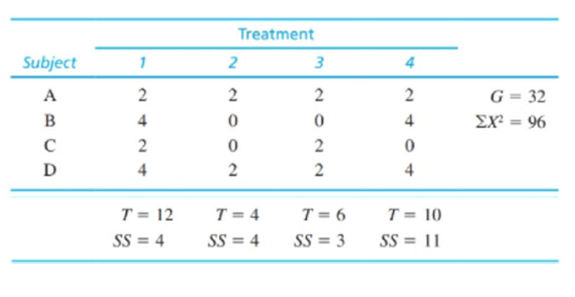Chapter 13.2, Problem 3LCAEssentials of Statistics for the B...

8th Edition
Frederick J Gravetter + 1 other
ISBN: 9781133956570

Solutions

Chapter
SectionEssentials of Statistics for the B...

8th Edition
Frederick J Gravetter + 1 other
ISBN: 9781133956570
Textbook Problem

For the following data, compute SSwithin treatments, SSbetween subjects and SSerror,To determine

To find: The SSwithintreatment,SSbetweensubjects and SSerror for the given question.

Explanation

Given info:

The data is

 Treatments G=32∑X2=96 Subject 1 2 3 4 A 1 2 2 2 B 4 0 0 4 C 2 0 2 0 D 4 2 2 4 T=12SS=4 T=4SS=4 T=6SS=3 T=10SS=11

Calculation:

Calculating the SSwithintreatment is:

SSwithintreatment=4+4+3+11=22

Thus, the SSwithintreatment is 22.

Now, the formula for SSbetweensubjects is:

SSbetweensubjects=P2kG2N

First find person total P values as:

For subject A: P=8

For subject B: P=8

For subject C: P=4

For subject D: P=12

Substituting these values we get:

SSbetweensubject=<

Still sussing out bartleby?

Check out a sample textbook solution.

See a sample solution

The Solution to Your Study Problems

Bartleby provides explanations to thousands of textbook problems written by our experts, many with advanced degrees!

Get Started

Find the mean for the following set of scores: 2, 7, 9, 4, 5, 3, 0, 6

Essentials of Statistics for The Behavioral Sciences (MindTap Course List)

Change 64 ft/s to mi/h.

Elementary Technical Mathematics

Write the sum in expanded form. 8. j=nn+3j2

Single Variable Calculus: Early Transcendentals, Volume I

Solve for x: ln(e + x) = 1. a) 0 b) 1 c) e d) ee e

Study Guide for Stewart's Single Variable Calculus: Early Transcendentals, 8th

Given: UT bisects WUV,WU=9,UV=12,WV=9 Find: WT

Elementary Geometry for College Students